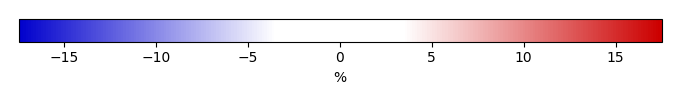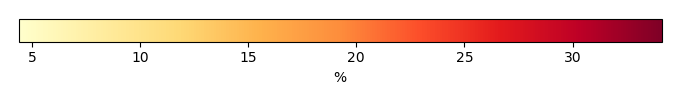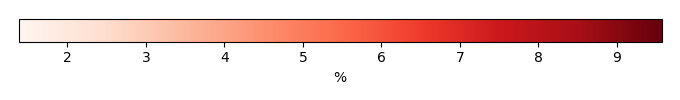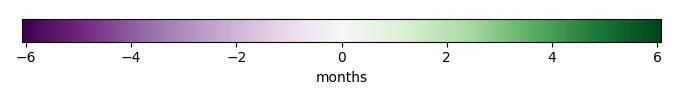# Mean State

Period Mean (original grids) [%]
Model Period Mean (intersection) [%]
Model Period Mean (complement) [%]
Benchmark Period Mean (intersection) [%]
Benchmark Period Mean (complement) [%]
Bias [%]
RMSE [%]
Phase Shift [months]
Bias Score 
RMSE Score 
Seasonal Cycle Score 
Spatial Distribution Score 
Interannual Variability Score 
Overall Score 
Benchmark [-] 77.9
CLM5PHSOFF [-] 70.0 70.1 77.8 80.3 -7.83 13.6 1.96 0.342 0.239 0.716 0.997 0.501 0.506
CLM5PHSON [-] 68.7 68.9 77.8 80.3 -8.28 14.1 1.93 0.336 0.234 0.722 0.997 0.484 0.501
Period Mean (original grids) [%]
Model Period Mean (intersection) [%]
Model Period Mean (complement) [%]
Benchmark Period Mean (intersection) [%]
Benchmark Period Mean (complement) [%]
Bias [%]
RMSE [%]
Phase Shift [months]
Bias Score 
RMSE Score 
Seasonal Cycle Score 
Spatial Distribution Score 
Interannual Variability Score 
Overall Score 
Benchmark [-] 53.6
CLM5PHSOFF [-] 51.4 50.8 53.2 77.5 -2.44 11.8 0.555 0.734 0.591 0.927 0.967 0.838 0.775
CLM5PHSON [-] 51.0 50.5 53.2 77.5 -2.52 11.8 0.548 0.733 0.592 0.929 0.969 0.837 0.775
Period Mean (original grids) [%]
Model Period Mean (intersection) [%]
Model Period Mean (complement) [%]
Benchmark Period Mean (intersection) [%]
Benchmark Period Mean (complement) [%]
Bias [%]
RMSE [%]
Phase Shift [months]
Bias Score 
RMSE Score 
Seasonal Cycle Score 
Spatial Distribution Score 
Interannual Variability Score 
Overall Score 
Benchmark [-] 64.2
CLM5PHSOFF [-] 62.5 62.3 63.5 77.8 -1.21 11.3 1.10 0.596 0.441 0.849 0.970 0.757 0.675
CLM5PHSON [-] 61.1 61.1 63.5 77.8 -1.12 11.5 1.17 0.595 0.435 0.837 0.971 0.749 0.670
Period Mean (original grids) [%]
Model Period Mean (intersection) [%]
Model Period Mean (complement) [%]
Benchmark Period Mean (intersection) [%]
Benchmark Period Mean (complement) [%]
Bias [%]
RMSE [%]
Phase Shift [months]
Bias Score 
RMSE Score 
Seasonal Cycle Score 
Spatial Distribution Score 
Interannual Variability Score 
Overall Score 
Benchmark [-] 77.6
CLM5PHSOFF [-] 89.0 88.9 77.1 83.4 11.8 17.9 2.90 0.111 0.0764 0.528 0.705 0.574 0.345
CLM5PHSON [-] 88.5 88.5 77.1 83.4 11.6 17.7 2.76 0.114 0.0792 0.549 0.727 0.555 0.350
Period Mean (original grids) [%]
Model Period Mean (intersection) [%]
Model Period Mean (complement) [%]
Benchmark Period Mean (intersection) [%]
Benchmark Period Mean (complement) [%]
Bias [%]
RMSE [%]
Phase Shift [months]
Bias Score 
RMSE Score 
Seasonal Cycle Score 
Spatial Distribution Score 
Interannual Variability Score 
Overall Score 
Benchmark [-] 84.2
CLM5PHSOFF [-] 84.8 84.8 85.0 81.2 -0.214 5.07 1.44 0.598 0.246 0.793 0.995 0.538 0.569
CLM5PHSON [-] 85.2 85.2 85.0 81.2 -0.688 5.23 1.42 0.587 0.237 0.797 0.998 0.516 0.562
Period Mean (original grids) [%]
Model Period Mean (intersection) [%]
Model Period Mean (complement) [%]
Benchmark Period Mean (intersection) [%]
Benchmark Period Mean (complement) [%]
Bias [%]
RMSE [%]
Phase Shift [months]
Bias Score 
RMSE Score 
Seasonal Cycle Score 
Spatial Distribution Score 
Interannual Variability Score 
Overall Score 
Benchmark [-] 72.3
CLM5PHSOFF [-] 82.8 82.8 72.2 78.9 10.6 18.6 2.07 0.319 0.187 0.694 0.975 0.582 0.491
CLM5PHSON [-] 82.6 82.6 72.2 78.9 10.4 17.9 2.16 0.321 0.199 0.684 0.974 0.573 0.492
Period Mean (original grids) [%]
Model Period Mean (intersection) [%]
Model Period Mean (complement) [%]
Benchmark Period Mean (intersection) [%]
Benchmark Period Mean (complement) [%]
Bias [%]
RMSE [%]
Phase Shift [months]
Bias Score 
RMSE Score 
Seasonal Cycle Score 
Spatial Distribution Score 
Interannual Variability Score 
Overall Score 
Benchmark [-] 61.3
CLM5PHSOFF [-] 65.7 65.9 61.0 71.3 4.95 16.2 2.01 0.545 0.320 0.696 0.995 0.761 0.606
CLM5PHSON [-] 65.2 65.4 61.0 71.3 5.02 16.2 1.97 0.540 0.322 0.705 0.994 0.756 0.606
Period Mean (original grids) [%]
Model Period Mean (intersection) [%]
Model Period Mean (complement) [%]
Benchmark Period Mean (intersection) [%]
Benchmark Period Mean (complement) [%]
Bias [%]
RMSE [%]
Phase Shift [months]
Bias Score 
RMSE Score 
Seasonal Cycle Score 
Spatial Distribution Score 
Interannual Variability Score 
Overall Score 
Benchmark [-] 69.0
CLM5PHSOFF [-] 64.4 64.0 68.3 79.0 -4.35 11.7 0.928 0.597 0.391 0.873 0.941 0.659 0.642
CLM5PHSON [-] 62.1 61.8 68.3 79.0 -4.10 11.5 0.920 0.608 0.395 0.875 0.943 0.655 0.645
Period Mean (original grids) [%]
Model Period Mean (intersection) [%]
Model Period Mean (complement) [%]
Benchmark Period Mean (intersection) [%]
Benchmark Period Mean (complement) [%]
Bias [%]
RMSE [%]
Phase Shift [months]
Bias Score 
RMSE Score 
Seasonal Cycle Score 
Spatial Distribution Score 
Interannual Variability Score 
Overall Score 
Benchmark [-] 78.8
CLM5PHSOFF [-] 89.0 89.0 78.5 84.9 10.4 16.4 2.77 0.152 0.0976 0.564 0.824 0.522 0.376
CLM5PHSON [-] 88.9 88.9 78.5 84.9 10.5 16.2 3.05 0.149 0.102 0.497 0.827 0.518 0.366
Period Mean (original grids) [%]
Model Period Mean (intersection) [%]
Model Period Mean (complement) [%]
Benchmark Period Mean (intersection) [%]
Benchmark Period Mean (complement) [%]
Bias [%]
RMSE [%]
Phase Shift [months]
Bias Score 
RMSE Score 
Seasonal Cycle Score 
Spatial Distribution Score 
Interannual Variability Score 
Overall Score 
Benchmark [-] 53.1
CLM5PHSOFF [-] 52.7 52.6 51.3 74.6 1.26 6.89 0.482 0.739 0.521 0.917 0.996 0.806 0.750
CLM5PHSON [-] 50.0 50.0 51.3 74.6 1.27 6.95 0.506 0.738 0.518 0.913 0.997 0.801 0.748
Period Mean (original grids) [%]
Model Period Mean (intersection) [%]
Model Period Mean (complement) [%]
Benchmark Period Mean (intersection) [%]
Benchmark Period Mean (complement) [%]
Bias [%]
RMSE [%]
Phase Shift [months]
Bias Score 
RMSE Score 
Seasonal Cycle Score 
Spatial Distribution Score 
Interannual Variability Score 
Overall Score 
Benchmark [-] 76.7
CLM5PHSOFF [-] 77.1 77.1 76.6 78.3 0.511 9.37 0.884 0.599 0.308 0.880 0.759 0.626 0.580
CLM5PHSON [-] 77.5 77.5 76.6 78.3 0.920 9.32 0.889 0.609 0.308 0.878 0.777 0.624 0.584
Period Mean (original grids) [%]
Model Period Mean (intersection) [%]
Model Period Mean (complement) [%]
Benchmark Period Mean (intersection) [%]
Benchmark Period Mean (complement) [%]
Bias [%]
RMSE [%]
Phase Shift [months]
Bias Score 
RMSE Score 
Seasonal Cycle Score 
Spatial Distribution Score 
Interannual Variability Score 
Overall Score 
Benchmark [-] 74.9
CLM5PHSOFF [-] 70.7 70.7 65.3 79.6 5.44 14.1 1.54 0.411 0.299 0.758 0.945 0.651 0.561
CLM5PHSON [-] 69.5 69.5 65.3 79.6 5.43 14.1 1.53 0.410 0.299 0.759 0.945 0.644 0.559
Period Mean (original grids) [%]
Model Period Mean (intersection) [%]
Model Period Mean (complement) [%]
Benchmark Period Mean (intersection) [%]
Benchmark Period Mean (complement) [%]
Bias [%]
RMSE [%]
Phase Shift [months]
Bias Score 
RMSE Score 
Seasonal Cycle Score 
Spatial Distribution Score 
Interannual Variability Score 
Overall Score 
Benchmark [-] 37.9
CLM5PHSOFF [-] 43.1 43.0 36.6 70.8 6.32 12.3 0.589 0.492 0.431 0.895 0.916 0.743 0.651
CLM5PHSON [-] 41.2 41.3 36.6 70.8 6.28 12.3 0.578 0.494 0.429 0.897 0.920 0.745 0.652
Period Mean (original grids) [%]
Model Period Mean (intersection) [%]
Model Period Mean (complement) [%]
Benchmark Period Mean (intersection) [%]
Benchmark Period Mean (complement) [%]
Bias [%]
RMSE [%]
Phase Shift [months]
Bias Score 
RMSE Score 
Seasonal Cycle Score 
Spatial Distribution Score 
Interannual Variability Score 
Overall Score 
Benchmark [-] 77.9
CLM5PHSOFF [-] 89.1 89.1 77.6 82.9 11.5 17.2 2.68 0.147 0.118 0.582 1.00 0.626 0.432
CLM5PHSON [-] 88.6 88.6 77.6 82.9 11.4 17.0 2.57 0.149 0.120 0.604 0.999 0.614 0.434
Period Mean (original grids) [%]
Model Period Mean (intersection) [%]
Model Period Mean (complement) [%]
Benchmark Period Mean (intersection) [%]
Benchmark Period Mean (complement) [%]
Bias [%]
RMSE [%]
Phase Shift [months]
Bias Score 
RMSE Score 
Seasonal Cycle Score 
Spatial Distribution Score 
Interannual Variability Score 
Overall Score 
Benchmark [-] 69.8
CLM5PHSOFF [-] 68.8 68.8 68.1 78.3 0.803 9.55 1.64 0.481 0.333 0.745 0.988 0.644 0.587
CLM5PHSON [-] 66.7 66.8 68.1 78.3 0.789 9.54 1.62 0.485 0.334 0.749 0.988 0.640 0.588
Period Mean (original grids) [%]
Model Period Mean (intersection) [%]
Model Period Mean (complement) [%]
Benchmark Period Mean (intersection) [%]
Benchmark Period Mean (complement) [%]
Bias [%]
RMSE [%]
Phase Shift [months]
Bias Score 
RMSE Score 
Seasonal Cycle Score 
Spatial Distribution Score 
Interannual Variability Score 
Overall Score 
Benchmark [-] 69.7
CLM5PHSOFF [-] 76.0 76.0 69.4 76.3 6.64 11.7 2.75 0.366 0.245 0.569 0.998 0.697 0.520
CLM5PHSON [-] 76.3 76.3 69.4 76.3 6.86 11.7 2.73 0.356 0.249 0.570 0.999 0.685 0.518
Period Mean (original grids) [%]
Model Period Mean (intersection) [%]
Model Period Mean (complement) [%]
Benchmark Period Mean (intersection) [%]
Benchmark Period Mean (complement) [%]
Bias [%]
RMSE [%]
Phase Shift [months]
Bias Score 
RMSE Score 
Seasonal Cycle Score 
Spatial Distribution Score 
Interannual Variability Score 
Overall Score 
Benchmark [-] 72.4
CLM5PHSOFF [-] 82.0 82.0 72.0 81.9 9.98 15.3 1.67 0.292 0.234 0.776 0.973 0.711 0.537
CLM5PHSON [-] 81.9 81.9 72.0 81.9 9.94 15.0 1.50 0.294 0.243 0.809 0.971 0.693 0.542
Period Mean (original grids) [%]
Model Period Mean (intersection) [%]
Model Period Mean (complement) [%]
Benchmark Period Mean (intersection) [%]
Benchmark Period Mean (complement) [%]
Bias [%]
RMSE [%]
Phase Shift [months]
Bias Score 
RMSE Score 
Seasonal Cycle Score 
Spatial Distribution Score 
Interannual Variability Score 
Overall Score 
Benchmark [-] 53.7
CLM5PHSOFF [-] 64.1 64.2 53.2 81.4 11.1 15.6 1.12 0.414 0.427 0.869 0.986 0.801 0.654
CLM5PHSON [-] 64.0 64.0 53.2 81.4 11.1 15.6 1.07 0.413 0.427 0.871 0.987 0.793 0.653
Period Mean (original grids) [%]
Model Period Mean (intersection) [%]
Model Period Mean (complement) [%]
Benchmark Period Mean (intersection) [%]
Benchmark Period Mean (complement) [%]
Bias [%]
RMSE [%]
Phase Shift [months]
Bias Score 
RMSE Score 
Seasonal Cycle Score 
Spatial Distribution Score 
Interannual Variability Score 
Overall Score 
Benchmark [-] 84.9
CLM5PHSOFF [-] 80.6 80.6 85.0 81.5 -4.39 10.5 1.29 0.445 0.261 0.833 0.888 0.542 0.538
CLM5PHSON [-] 80.2 80.2 85.0 81.5 -4.70 10.9 1.30 0.431 0.252 0.831 0.894 0.520 0.530
Period Mean (original grids) [%]
Model Period Mean (intersection) [%]
Model Period Mean (complement) [%]
Benchmark Period Mean (intersection) [%]
Benchmark Period Mean (complement) [%]
Bias [%]
RMSE [%]
Phase Shift [months]
Bias Score 
RMSE Score 
Seasonal Cycle Score 
Spatial Distribution Score 
Interannual Variability Score 
Overall Score 
Benchmark [-] 70.7
CLM5PHSOFF [-] 72.1 71.9 70.4 79.2 1.49 13.0 1.32 0.462 0.322 0.826 0.728 0.718 0.563
CLM5PHSON [-] 71.7 71.5 70.4 79.2 1.59 13.1 1.36 0.462 0.318 0.819 0.724 0.716 0.560
Period Mean (original grids) [%]
Model Period Mean (intersection) [%]
Model Period Mean (complement) [%]
Benchmark Period Mean (intersection) [%]
Benchmark Period Mean (complement) [%]
Bias [%]
RMSE [%]
Phase Shift [months]
Bias Score 
RMSE Score 
Seasonal Cycle Score 
Spatial Distribution Score 
Interannual Variability Score 
Overall Score 
Benchmark [-] 65.5
CLM5PHSOFF [-] 64.6 64.8 63.7 81.7 1.00 12.3 0.912 0.478 0.375 0.869 0.969 0.635 0.617
CLM5PHSON [-] 62.9 63.1 63.7 81.7 0.944 12.3 0.915 0.480 0.372 0.868 0.972 0.633 0.616

# Temporally integrated period mean

BENCHMARK MEANMODEL MEANBIASBIAS SCORERMSERMSE SCOREBENCHMARK INTERANNUAL VARIABILITYMODEL INTERANNUAL VARIABILITYINTERANNUAL VARIABILITY SCOREBENCHMARK MAX MONTHMODEL MAX MONTHDIFFERENCE IN MAX MONTHSEASONAL CYCLE SCORESPATIAL TAYLOR DIAGRAMMODEL COLORS# Spatially integrated regional mean

MODEL COLORSREGIONAL MEANANNUAL CYCLEMONTHLY ANOMALYANNUAL CYCLE# All Models

BenchmarkCLM5PHSOFFCLM5PHSON# Data Information

creation_date: Wed Dec 2 12:03:21 PST 2015

Conventions: Please contact Prof. James Randerson (Email: jranders@uci.edu) or Dr. Mingquan Mu (mmu@uci.edu) for any question

source_file: This product is generated from monthly ERA interim observations

title: derived ERA interim

Approach: I read the monthly 2m dewpoint temperature and 2m surface air temperature from original file, and calculated 2m relative humidity using the way by John Dutton (Ceaseless Wind, John Dutton. McGraw-Hill, 1976. pp 273-274). The final data were saved by each month and each year.

Temporal resolution: monthly

General information: This product was derived from ERA interim Dataset (1979-present).

Spatial resolution: 0.5x0.5, 1x1 and 2.5x2.5 degree